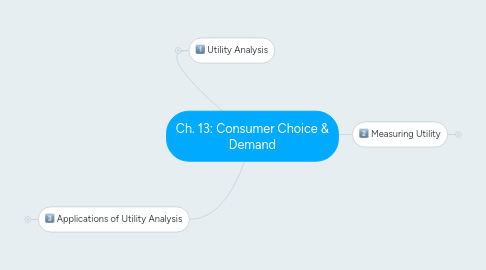# Ch. 13: Consumer Choice & Demand

Get Started. It's FreeCh. 13: Consumer Choice & Demand## 1. Utility Analysis

### 1.1. Tastes & preferences

1.1.1. Your likes & dislikes in consumption

### 1.2. The Law of Diminishing Marginal Utility

1.2.1. Total utility

1.2.1.1. is total satisfaction you derive from consumption

1.2.2. Marginal utility

1.2.2.1. change in total utility

1.2.2.2. from a 1 unit change in consumption

1.2.3. Law of Marginal Utility

1.2.3.1. The more of a good a person consumes,

1.2.3.2. the smaller the increase in total utility

## 2. Measuring Utility

### 2.1. Units of Utility

2.1.1. Develop numerical values

2.1.2. Each person has a uniquely subjective utility scale

### 2.2. Maximizing utility without scarcity

2.2.1. If a good is free,

2.2.1.1. you increase consumption as long as marginal utility is positive.

### 2.3. Maximizing utility with scacity

2.3.1. Must consider:

2.3.1.1. Limited supply

2.3.1.2. Income

### 2.5. Relationship with Law of Demand

2.5.1. Can use points at different price levels to construct a demand curve.

## 3. Applications of Utility Analysis

### 3.1. Consumer surplus

3.1.1. Difference between the most a consumer would pay and what is actually paid

3.1.2. Marginal valuation

3.1.2.1. \$ value of the marginal utility derived from consuming each additional unit of a good

3.1.3. On graph, it is the area under demand curve but above price

### 3.2. Market demand & consumer surplus

3.2.1. Market demand is the horizontal summation of individual demand curves

3.2.2. Consumer surplus can be illustrated for individuals as well as markets

### 3.3. Role of time in demand

3.3.1. Consumption has both time and money price

3.3.2. Some people willing to pay more to get things quicker

3.3.2.1. Convenience stores

3.3.2.2. Amazon Prime